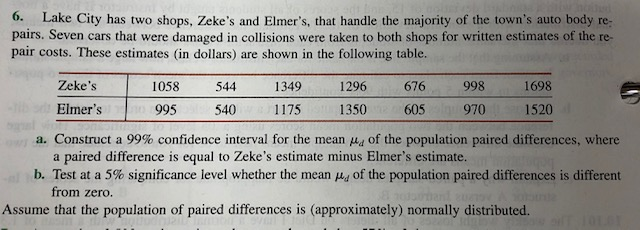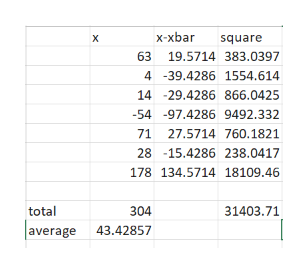# 6.Lake City has two shops, Zeke's and Elmer's, that handle the majority of the town's auto body re-pairs. Seven cars that were damaged in collisions were taken to both shops for written estimates of the re-pair costs. These estimates (in dollars) are shown in the following table.Zeke's1058544134912966769981698Elmer's152099554011751350605970a. Construct a 99% confidence interval for the mean of the population paired differences, wherea paired difference is equal to Zeke's estimate minus Elmer's estimate.b. Test at a 5% significance level whether the mean 4 of the population paired differences is differentfrom zero.Assume that the population of paired differences is (approximately) normally distributed.

Question
10 views

3. Can a test for the difference in proportions from Chapter 10 and a chi-square test of independence/association be utilized to analyze data from a 2x2 contingency table? Provide an example.

see the attached photo for an example of ch10 problem.help_outlineImage Transcriptionclose6. Lake City has two shops, Zeke's and Elmer's, that handle the majority of the town's auto body re- pairs. Seven cars that were damaged in collisions were taken to both shops for written estimates of the re- pair costs. These estimates (in dollars) are shown in the following table. Zeke's 1058 544 1349 1296 676 998 1698 Elmer's 1520 995 540 1175 1350 605 970 a. Construct a 99% confidence interval for the mean of the population paired differences, where a paired difference is equal to Zeke's estimate minus Elmer's estimate. b. Test at a 5% significance level whether the mean 4 of the population paired differences is different from zero. Assume that the population of paired differences is (approximately) normally distributed. fullscreen
check_circle

Step 1

Given:

In a lake city there are two shops, Zeke’s and Elmer’s that handle the majority of the town’s auto body repairs.

Number of cars: 7

Zeke’s estimation: 1058 544 1349 1296 676 998 1698

Elmer’s estimation: 995 540 1175 1350 605 970 1520

Step 2

a)

Construct a 99% C.I for the mean of the population differences

They give that the population difference is equal to (Zeke’s estimate – Elmer’s estimate)

Hence, difference is 63 4 174 -54 71 28 178

Mean of the difference is 43.4286.

Standard deviation is 72.3460.help_outlineImage Transcriptionclosex-xbar X square 63 19.5714 383.0397 4 -39.4286 1554.614 14 -29.4286 866.0425 -54 -97.4286 9492.332 71 27.5714 760.1821 28 -15.4286 238.0417 178 134.5714 18109.46 31403.71 total 304 average 43.42857 fullscreen
Step 3

### Want to see the full answer?

See Solution

#### Want to see this answer and more?

Solutions are written by subject experts who are available 24/7. Questions are typically answered within 1 hour.*

See Solution
*Response times may vary by subject and question.
Tagged in

### Hypothesis Testing